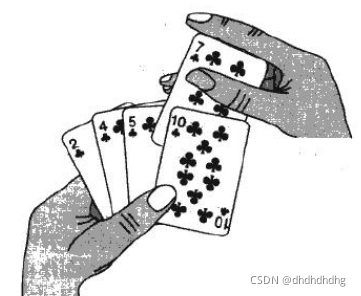﻿ Java中七种排序算法总结分析_java_脚本之家
javaJava中七种排序算法总结分析

一、插入排序

1.基本思想2.直接插入排序

1.第一次排序先将第一个元素看成是已排序区间，将其内容保存起来
2.待排序区间是其后面的所有元素，从其后面第一个开始，依次和已排序区间里的元素比较（这里的比较是从后向前比较，也就是从其前一个元素比较到0号位置的元素）
3.如果小于已排序区间中的元素，则将该位置向后挪一位
4.直到找到第一个比其小的元素，此时其后面的所有元素都是比它大的，且都向后挪了一位，这时其后面的位置是空的，将该元素放进来即可。
5.之后取第二个，第三个…第i个依次这样比较即可，即循环起来。其中每次[0,i)是已排序区间，[i,size)是待排序区间。public static void insertSort(int[] array){
int j=0;
//i位置与之前所有下标从后往前进行比较，该位置大于i则继续往前，小于则插入到后边
//[0,bound)已排序区间
//[bound,size)待排序区间
for(int bound=1;bound<array.length;bound++) {
int temp = array[bound];
//先取已排序区间的最后一个，依次往前进行比较
for (j = bound - 1; j >= 0; j--) {
//如果temp小于，则将元素往后移一位
if (array[j]>temp) {
array[j + 1] = array[j];
} else {
break;
}
}
//找到合适位置，填充
array[j + 1] = temp;
}
}

3.希尔排序(缩小增量排序)

1.设置gap值（每个组内元素之间间隔）
2.在每个组内进行直接插入排序
3.缩小gap值（这里我按照2倍缩小）
4.gap为1时排序完毕public static void shellSort(int[] array){
int gap= array.length/2;
while(gap>0){
int j=0;
//i位置与之前所有下标从后往前进行比较，该位置大于i则继续往前，小于则插入到后边
for(int bound=gap;bound<array.length;bound+=gap) {
int temp = array[bound];
for (j = bound - gap; j >= 0; j-=gap) {
if (array[j]>temp) {
array[j + gap] = array[j];
} else {
break;
}
}
array[j + gap] = temp;
}
gap=gap/2;
}
}

二、选择排序

2.直接选择排序

1.先记录下标为0元素为最小值
2.从下标为1开始往后遍历，遇到比最小值还小的就标记起来，遍历完成即找到最小值
3.将找到的最小值与下标为0的元素进行交换
4.此时[0,1）为已排序区间，即不再动，[1,size)是待排序区间
5.从下标为1继续上面的操作，即循环起来
6.循环到最后只剩一个元素结束，排序完成public static void selectSort(int[] nums) {
//每次都和第一个元素相比，如果小于则和一个元素则交换
for(int bound=0;bound<nums.length-1;bound++){
//先记录最小值为第一个元素
int min=bound;
//从其后一个开始遍历，找出后面所有元素中的最小值
for(int i=bound+1;i<nums.length;i++){
if(nums[min]>nums[i]){
min=i;
}
}
//将最小值与待排序区间第一个进行交换
swap(nums,bound,min);
}
}

3.堆排序

1.创建一个大堆（升序）

2.将根结点与最后一个结点进行交换，此时最后一个元素一定是所有元素中最大的
3.将size–
4.将堆继续调整好形成一个大堆，继续上面的操作，即循环起来
5.最终排序完成//写一个交换函数
private static void swap(int[] array,int parent,int child){
int temp=array[child];
array[child]=array[parent];
array[parent]=temp;
}
//排升序建大堆
//向下调整，上篇博客提到了
private static void shiftDown(int[] array,int parent,int size){
int child=parent*2+1;
while(child<size){
if(child+1<size&&array[child+1]>array[child]){
child+=1;
}
if(array[parent]<array[child]){
swap(array,parent,child);
parent=child;
child=parent*2+1;
}else{
return;
}
}
}
//堆排序
public static void heapSort(int[] array){
//创建一个堆
int size=array.length;
for(int root=(size-2)/2;root>=0;root--){
shiftDown(array,root,size);
}
while(size>1){
//将第一个和最后一个元素进行交换
swap(array,0,size-1);
//交换完成向下调整
size--;
shiftDown(array,0,size);
}
}

三、交换排序

2.冒泡排序

1.从0号位置开始，将元素和他后面的元素比较，如果大于则交换
2.当遍历完成，最大的元素肯定在数组的最后
3.之后再比较时，最后一个元素就是已排序区间，不再参与比较
4.重复上述步骤，即循环起来public static void bubbleSort(int[] nums) {
//外层循环控制循环次数
for(int num=1;num<nums.length;num++){
//内层循环控制交换哪两个元素
for(int i=0;i<nums.length-num;i++){
if(nums[i+1]<nums[i]){
swap(nums,i+1,i);
}
}
}
}

3.快速排序（递归与非递归）

public static void quickSort(int[] array,int left,int right){
if((right-left)<=1){
return;
}
//按照基准值对array数组的[left,right)区间中的元素进行划分
int div=partition3(array,left,right);

//划分成功后以div为边界形成了左右两部分[left,div)和[div+1,right)
//递归排[left,div)
quickSort(array,left,div);

//递归排[div+1,right)
quickSort(array,div+1,right);
}

1.Hoare版

1.这里我们取最右侧为基准值
2.设置begin和end用来标记，让begin从左往右走，end从右往左走
3.当begin小于end时，因为我们取得基准值为最右侧，所以应先让begin从左往右走，找到比基准值大的元素就停下来
4.这时让end从右往左走，找到比基准值小的元素就停下来，两者交换位置
5.循环起来
6.当循环结束时，两个最终走到了同一个位置，将其和基准值进行交换private static int partition1(int[] array,int left,int right){
int temp=array[right-1];
//begin从左往右找
int begin=left;
//end从右往左找
int end=right-1;
while(begin<end){
//让begin从前往后找比temp大的元素，找到之后停下来
while(array[begin]<=temp&&begin<end){
begin++;
}
//让end从后往前找比temp小的元素，找到之后停下来
while(array[end]>=temp&&begin<end){
end--;
}
//当两个在同一个位置时，不需要交换，减少交换次数
if(begin!=end){
swap(array,begin,end);
}
}
if(begin!=right-1){
swap(array,begin,right-1);
}
return begin;
}

2.挖坑法

1.先将基准值（这里是最右侧元素）放在临时变量temp中，这里就形成第一个坑位
2.设置begin和end用来标记，让begin从左往右走，end从右往左走
3.当begin小于end时，让begin从左往右走，找到比temp大的值，去填end的坑，同时begin这里形成一个坑位
4.然后让end从右往左走，找到比temp小的值，去填begin的坑，同时end这里形成了一个新的坑位
5.就这样一直循环，互相填坑，直到不满足循环条件
6.这时begin和end在同一个坑位，且是最后一个坑位，使用基准值填充private static int partition2(int[] array,int left,int right){
int temp=array[right-1];
int begin=left;
int end=right-1;
while(begin<end){
//让begin从前往后找比基准值大的元素
while(array[begin]<=temp&&begin<end){
begin++;
}
if(begin!=end){
//begin位置找到了一个比temp大的元素，填end的坑
array[end]=array[begin];
//begin位置形成了一个新的坑位
}
//让end从后往前找比基准值小的元素
while(array[end]>=temp&&begin<end){
end--;
}
if(begin!=end){
//end位置找到了一个比temp小的元素，填begin的坑
array[begin]=array[end];
//end位置形成了一个新的坑位
}
}
//begin和end位置是最后的一个坑位
//这个坑位使用基准值填充
array[begin]=temp;
return begin;
}

3.前后标记法（难理解）

1.采用cur和prev来标记，两者都是从左往右走，不一样的是，最开始cur在0号位置，prev在cur前一个位置上
2.当遇到比temp大的元素时，prev不动，cur向前走一步，当两者一前一后的状态时，cur也会往前走一步，而prev不动
3.这样两者逐渐拉开差距，此时遇到比基准值小的元素，cur中的元素就会与prev中的元素交换
4.走到最后prev的下一个元素与基准值位置交换private static int partition3(int[] array,int left,int right){
int temp=array[right-1];
int cur=left;
int prev=cur-1;
//让cur从前往后找比基准值小的元素
while(cur<right){
if(array[cur]<temp&&++prev!=cur){
swap(array,cur,prev);
}
cur++;
}
//将基准值的位置放置好
if(++prev!=right-1){
swap(array,prev,right-1);
}
return prev;
}

4.快速排序优化

1.三数取中法选temp

private static int getIndexOfMid(int[] array,int left,int right){
int mid=left+((right-left)>>1);
if(array[left]<array[right-1]){
if(array[mid]<array[left]){
return left;
}else if(array[mid]>array[right-1]){
return right-1;
}else{
return mid;
}
}

int index=getIndexOfMid(array,left,right);
if(index!=right-1){
swap(array,index,right-1);
}

2.递归到小的子区间时，可以考虑使用插入排序

5.快速排序非递归

public static void quickSortNor(int[] array){
Stack<Integer> s=new Stack<>();
s.push(array.length);
s.push(0);
while(!s.empty()){
int left=s.pop();
int right=s.pop();
if((right-left)<47){
insertSortQuick(array,left,right);
}else{
int div=partition1(array,left,right);
//先压入基准值的右半侧
s.push(right);
s.push(div+1);
//再压入基准值的左半侧
s.push(div);
s.push(left);
}
}
}

四、归并排序（递归与非递归）private static void mergeData(int[] array,int left,int mid,int right,int[] temp){
int begin1=left,end1=mid;
int begin2=mid,end2=right;
int index=left;
while(begin1<end1&&begin2<end2){
//依次比较每一位上的元素，小的放入temp同时下标后移一位
if(array[begin1]>array[begin2]){
temp[index++]=array[begin2++];
}else {
temp[index++]=array[begin1++];
}
}
//看两个数组哪个还没有遍历完成，直接顺次放入temp数组的后面
while(begin1<end1){
temp[index++] = array[begin1++];
}
while(begin2<end2){
temp[index++]=array[begin2++];
}
}

1.先对待排序区间进行均分为两个
2.使用递归，直到均分为每个区间只剩下一个元素时，每两个使用二路归并
3.将两个有序数组合并为一个有序数组，将结果保存在temp中
4.最终temp保存的就是排序完成的数组，再拷贝回原数组中

private static void mergeSort(int[] array,int left,int right,int[] temp){
if((right-left>1)){
//先对[left,right)区间中的元素进行均分
int mid=left+((right-left)>>1);
//[left,mid)
mergeSort(array,left,mid,temp);
//[mid,right)
mergeSort(array,mid,right,temp);
//二路归并
mergeData(array,left,mid,right,temp);
//将temp中的元素拷贝回原数组中
System.arraycopy(temp,left,array,left,right-left);
}
}

public static void mergeSortNor(int[] array){
int size=array.length;
int gap=1;
while(gap<size){
for(int i=0;i<size;i+=2*gap){
//划分好要归并的区域，第一次每个区间只有一个元素
//[left,mid)
int left=i;
int mid=left+gap;
//[mid,right)
int right=mid+gap;
if(mid>size){
mid=size;
}
if(right>size){
right=size;
}
int[] temp=new int[size];
//二路归并
mergeData(array,left,mid,right,temp);
//将temp中的元素拷贝回原数组中
System.arraycopy(temp,left,array,left,right-left);
}
//gap值翻二倍，继续归并
gap<<=1;
}
}